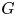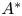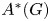Home
Hostname: page-component-59b7f5684b-569ts Total loading time: 1.619 Render date: 2022-09-28T07:41:10.564Z Has data issue: true Feature Flags: { "shouldUseShareProductTool": true, "shouldUseHypothesis": true, "isUnsiloEnabled": true, "useRatesEcommerce": false, "displayNetworkTab": true, "displayNetworkMapGraph": false, "useSa": true } hasContentIssue trueCompositio Mathematica

# Hopf-theoretic approach to motives of twisted flag varieties

Published online by Cambridge University Press:  29 April 2021

## Abstract

Let$G$ be a split semisimple algebraic group over a field and let$A^*$ be an oriented cohomology theory in the Levine–Morel sense. We provide a uniform approach to the$A^*$-motives of geometrically cellular smooth projective$G$-varieties based on the Hopf algebra structure of$A^*(G)$. Using this approach, we provide various applications to the structure of motives of twisted flag varieties.

## MSC classification

Type
Research Article
Information
Compositio Mathematica , May 2021 , pp. 963 - 996
© The Author(s) 2021

## Access options

Get access to the full version of this content by using one of the access options below. (Log in options will check for institutional or personal access. Content may require purchase if you do not have access.)

## Footnotes

The first author was supported by Laboratory of Modern Algebra and Applications, St. Petersburg State University, via a grant of the government of the Russian Federation for the state support of scientific research carried out under the supervision of leading scientists, agreement 14.W03.31.0030 dated 15.02.2018, by Young Russian Mathematics award and by RFBR grant 18-31-20044. The second author acknowledges the support of the SPP 1786 ‘Homotopy theory and algebraic geometry’ (DFG).

## References

Brosnan, P., On motivic decompositions arising from the method of Białynicki-Birula, Invent. Math. 161 (2005), 91111.CrossRefGoogle Scholar
Calmès, B., Neshitov, A. and Zainoulline, K., Relative equivariant motives and modules, Can. J. Math. 73 (2021), 131159.CrossRefGoogle Scholar
Calmès, B., Petrov, V. and Zainoulline, K., Invariants, torsion indices and oriented cohomology of complete flags, Ann. Sci. École Norm. Supér. 46 (2013), 405448.CrossRefGoogle Scholar
Chernousov, V., Gille, S. and Merkurjev, A., Motivic decomposition of isotropic projective homogeneous varieties, Duke Math. J. 126 (2005), 137159.CrossRefGoogle Scholar
Chernousov, V. and Merkurjev, A., Motivic decomposition of projective homogeneous varieties and the Krull–Schmidt theorem, Transf. Groups 11 (2006), 371386.CrossRefGoogle Scholar
Demazure, M., Invariants symétriques entiers des groupes de Weyl et torsion, Invent. Math. 21 (1973), 287301.CrossRefGoogle Scholar
Demazure, M., Désingularisation des variétés de Schubert généralisées, Ann. Sci. Éc. Norm. Supér. 7 (1974), 5388.CrossRefGoogle Scholar
Duan, H. and Zhao, X., A unified formula for Steenrod operations on flag manifolds, Compos. Math. 143 (2007), 257270.CrossRefGoogle Scholar
Duan, H. and Zhao, X., The Chow rings of generalized Grassmannians, Found. Comput. Math. 10 (2010), 245274.CrossRefGoogle Scholar
Edidin, D. and Graham, W., Characteristic classes in the Chow ring, J. Algebr. Geom. 6 (1997), 431443.Google Scholar
Edidin, D. and Graham, W., Equivariant intersection theory (with an appendix by A. Vistoli: The Chow ring of$\mathcal {M}_2$), Invent. Math. 131 (1998), 595634.CrossRefGoogle Scholar
Elman, R., Karpenko, N. and Merkurjev, A., The algebraic and geometric theory of quadratic forms, Colloquium Publications, vol. 56 (American Mathematical Society, Providence, RI, 2008).CrossRefGoogle Scholar
Garibaldi, S., Petrov, V. and Semenov, N., Shells of twisted flag varieties and the Rost invariant, Duke Math. J. 165 (2016), 285339.CrossRefGoogle Scholar
Garibaldi, S. and Semenov, N., Degree$5$ invariant of$\mathrm {E}_8$, Int. Math. Res. Not. 2010 (2010), 37463762.Google Scholar
Gille, S. and Vishik, A., Rost nilpotence and free theories, Doc. Math. 23 (2018), 16351657.Google Scholar
Gille, S. and Zainoulline, K., Equivariant pretheories and invariants of torsors, Transf. Groups 17 (2012), 471498.CrossRefGoogle Scholar
Ishitoya, K., Kono, A. and Toda, H., Hopf algebra structure of mod$2$ cohomology of simple Lie groups, Publ. Res. Inst. Math. Sci. 12 (1976/77), 141167.CrossRefGoogle Scholar
Kac, V., Torsion in cohomology of compact Lie groups and Chow rings of reductive algebraic groups, Invent. Math. 80 (1985), 6979.10.1007/BF01388548CrossRefGoogle Scholar
Karpenko, N., On the first Witt index of quadratic forms, Invent. Math. 153 (2003), 455462.CrossRefGoogle Scholar
Karpenko, N., Holes in$I^n$, Ann. Sci. Éc. Norm. Supér. (4) 37 (2004), 9731002.CrossRefGoogle Scholar
Karpenko, N., Upper motives of algebraic groups and incompressibility of Severi–Brauer varieties, J. Reine Angew. Math. 677 (2013), 179198.Google Scholar
Karpenko, N. and Zhykhovich, M., Isotropy of unitary involutions, Acta Math. 211 (2013), 227253.CrossRefGoogle Scholar
Kono, A. and Mimura, M., Cohomology operations and the Hopf algebra structures of the compact, exceptional Lie groups$E_7$ and$E_8$, Proc. Lond. Math. Soc. 35 (1977), 345358.CrossRefGoogle Scholar
Levine, M. and Morel, F., Algebraic cobordism (Springer, Berlin, 2007).Google Scholar
Malaschonok, G., Fast generalized Bruhat decomposition, in Computer Algebra in Scientific Computing: 12th International Workshop, CASC 2010, eds V. P. Gerdt, W. Koepf, E. W. Mayr, and E. V. Vorozhtsov, Lecture Notes in Computer Science, vol. 6244 (Springer, Berlin, Heidelberg, 2010), 194–202.Google Scholar
Manin, Y., Correspondences, motives and monoidal transformations, Math. USSR Sbornik 6 (1968), 439470.CrossRefGoogle Scholar
May, J. P. and Zabrodsky, A.,$H^*\operatorname {\mathrm {Spin}}(n)$ as a Hopf algebra, J. Pure Appl. Algebra 10 (1977/78), 193200.CrossRefGoogle Scholar
Merkurjev, A., Comparison of the equivariant and the standard$K$-theory of algebraic varieties, Algebr. Anal. 9 (1997), 175214; translation in St. Petersburg Math. J. 9 (1998), 815–850.Google Scholar
Milnor, J. and Moore, J., On the structure of Hopf algebras, Ann. Math. 81 (1965), 211264.CrossRefGoogle Scholar
Mimura, M. and Toda, H., Topology of Lie groups, I and II, translated from the 1978 Japanese edition by the authors. Translations of Mathematical Monographs, vol. 91 (American Mathematical Society, Providence, RI, 1991).Google Scholar
Nenashev, A. and Zainoulline, K., Oriented cohomology and motivic decompositions of relative cellular spaces, J. Pure Appl. Algebra 205 (2006), 323340.CrossRefGoogle Scholar
Neshitov, A., Petrov, V., Semenov, N. and Zainoulline, K., Motivic decompositions of twisted flag varieties and representations of Hecke-type algebras, Adv. Math. 340 (2018), 791818.CrossRefGoogle Scholar
Nikolenko, S., Semenov, N. and Zainoulline, K., Motivic decomposition of anisotropic varieties of type$\mathrm {F}_4$ into generalized Rost motives, J. K-Theory 3 (2009), 85102.CrossRefGoogle Scholar
Panin, I., On the algebraic$K$-theory of twisted flag varieties, K-Theory 8 (1994), 541585.CrossRefGoogle Scholar
Petrov, V. and Semenov, N., Generically split projective homogeneous varieties, Duke Math. J. 152 (2010), 155173.CrossRefGoogle Scholar
Petrov, V. and Semenov, N., Generically split projective homogeneous varieties. II, J. K-Theory 10 (2012), 18.CrossRefGoogle Scholar
Petrov, V. and Semenov, N., Rost motives, affine varieties, and classifying spaces, J. Lond. Math. Soc. 95 (2017), 895918.CrossRefGoogle Scholar
Petrov, V., Semenov, N. and Zainoulline, K.,$J$-invariant of linear algebraic groups, Ann. Sci. Éc. Norm. Supér. 41 (2008), 10231053.CrossRefGoogle Scholar
Plotkin, E., Semenov, A. and Vavilov, N., Visual basic representations: an atlas, Internat. J. Algebra Comput. 8 (1998), 6195.CrossRefGoogle Scholar
Primozic, E., Motivic Steenrod operations in characteristic$p$, Forum Math. Sigma 8 (2020), article no. e52.CrossRefGoogle Scholar
Quéguiner-Mathieu, A., Semenov, N. and Zainoulline, K., The$J$-invariant, Tits algebras and triality, J. Pure Appl. Algebra 216 (2012), 26142628.CrossRefGoogle Scholar
Quillen, D., Higher algebraic K-theory. I, Lecture Notes in Mathematics, vol. 341 (Springer, Berlin, 1973).CrossRefGoogle Scholar
Ravenel, D., Complex cobordism and stable homotopy groups of spheres, second edition (AMS Chelsea Publishing, Providence, RI, 2004).Google Scholar
Rost, M., The motive of a Pfister form. Preprint (1998), http://www.math.uni-bielefeld.de/~rost.Google Scholar
Sechin, P. and Semenov, N., Applications of the Morava$K$-theory to algebraic groups, Ann. Sci. Éc. Norm. Supér., to appear (2020). Preprint (2018), arXiv:1805.09059.Google Scholar
Semenov, N., Hasse diagrams and motives of projective homogeneous varieties, J. Math. Sci. 140 (2007), 737741.CrossRefGoogle Scholar
Semenov, N., Motivic construction of cohomological invariants, Comment. Math. Helv. 91 (2016), 163202.CrossRefGoogle Scholar
Semenov, N. and Zhykhovich, M., Integral motives, relative Krull–Schmidt principle, and Maranda-type theorems, Math. Ann. 363 (2015), 6175.CrossRefGoogle Scholar
Swan, R.,$K$-theory of quadric hypersurfaces, Ann. Math. 122 (1985), 113153.CrossRefGoogle Scholar
Tits, J., Classification of algebraic semisimple groups, in Algebraic groups and discontinuous subgroups, Proceedings of Symposia in Pure Mathematics (American Mathematical Society, Providence, RI, 1966), 3362.CrossRefGoogle Scholar
Vishik, A., Motives of quadrics with applications to the theory of quadratic forms, in Geometric methods in the algebraic theory of quadratic forms, Lecture Notes in Mathematics, vol. 1835 (Springer, Berlin, 2004), 25101.CrossRefGoogle Scholar
Vishik, A., On the Chow groups of quadratic Grassmannians, Doc. Math. 10 (2005), 111130.Google Scholar
Vishik, A., Fields of u-invariant 2r + 1, in Algebra, arithmetic and geometry: In Honor of Yu. I. Manin, Vol. II (Birkhäuser, Boston, MA, 2009), 661–685.Google Scholar
Vishik, A., Excellent connections in the motives of quadrics, Ann. Sci. Éc. Norm. Supér. 44 (2011), 183195.CrossRefGoogle Scholar
Vishik, A. and Yagita, N., Algebraic cobordisms of a Pfister quadric, J. Lond. Math. Soc. 76 (2007), 586604.CrossRefGoogle Scholar
Yagita, N., Algebraic cobordism of simply connected Lie groups, Math. Proc. Cambridge Philos. Soc. 139 (2005), 243260.CrossRefGoogle Scholar

# Save article to Kindle

To save this article to your Kindle, first ensure coreplatform@cambridge.org is added to your Approved Personal Document E-mail List under your Personal Document Settings on the Manage Your Content and Devices page of your Amazon account. Then enter the ‘name’ part of your Kindle email address below. Find out more about saving to your Kindle.

Note you can select to save to either the @free.kindle.com or @kindle.com variations. ‘@free.kindle.com’ emails are free but can only be saved to your device when it is connected to wi-fi. ‘@kindle.com’ emails can be delivered even when you are not connected to wi-fi, but note that service fees apply.

Find out more about the Kindle Personal Document Service.

Hopf-theoretic approach to motives of twisted flag varieties
Available formats
×

# Save article to Dropbox

To save this article to your Dropbox account, please select one or more formats and confirm that you agree to abide by our usage policies. If this is the first time you used this feature, you will be asked to authorise Cambridge Core to connect with your Dropbox account. Find out more about saving content to Dropbox.

Hopf-theoretic approach to motives of twisted flag varieties
Available formats
×

# Save article to Google Drive

To save this article to your Google Drive account, please select one or more formats and confirm that you agree to abide by our usage policies. If this is the first time you used this feature, you will be asked to authorise Cambridge Core to connect with your Google Drive account. Find out more about saving content to Google Drive.

Hopf-theoretic approach to motives of twisted flag varieties
Available formats
×
×